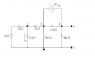# Finding Rth of a basic circuit

• Engineering
• orangeincup
In summary, the conversation is about finding the Thevenin equivalent with respect to the terminals a,b. The person is trying to calculate the Thevenin resistance (Rth) by removing the sources from the circuit and using the node voltage/mesh current method. However, they are unsure if they should short the voltage source or open the current source. They also mention using the parallel resistor formula to calculate Rth, but are not sure if they should ignore some resistors. It is also noted that there is a dependent current source in the circuit.

## Homework Statement

Determine the Thevenin equivalent with respect to the terminals a,b.

Rth=Voc/Isc

## The Attempt at a Solution

Right now I'm just trying to calculate Rth by removing the sources from the circuit. When I calculated the Rth using the node voltage/mesh current method I calculated it to be 20 kΩ.

So right now my idea is I have to short out the voltage source and open the current source.

I think(?) this means the 2kΩ, 20kΩ, and 5kΩ(maybe not) would be canceled out and the rest of the circuit would be intact.

So I calculated Rth based on the rest...

(40kΩ+10kΩ)||50kΩ=25kΩ

Can someone explain what I did wrong?

I also tried doing
(10kΩ+50kΩ)||20kΩ+5kΩ which gave me an answer of 20kΩ but I do not think I can add the 5kΩ resistor to the 20kΩ resistor in that order.

#### Attachments

•rth2.png
2 KB · Views: 577
I think(?) this means the 2kΩ, 20kΩ, and 5kΩ(maybe not) would be canceled out and the rest of the circuit would be intact.
shorting the voltage source means the 2k and 20k resistors are in parallel.

(40kΩ+10kΩ)||50kΩ=25kΩ

(25kΩ+5kΩ)||20kΩ=12kΩ

12kΩ||2kΩ=1.74kΩ

Am I suppose to ignore some of the resistors due to shorting the current source? What does opening it do to the 10kΩ or the 40kΩ resistor?

Am I suppose to open the voltage source and short the circuit source or vise versa?

It's a dependent current source.